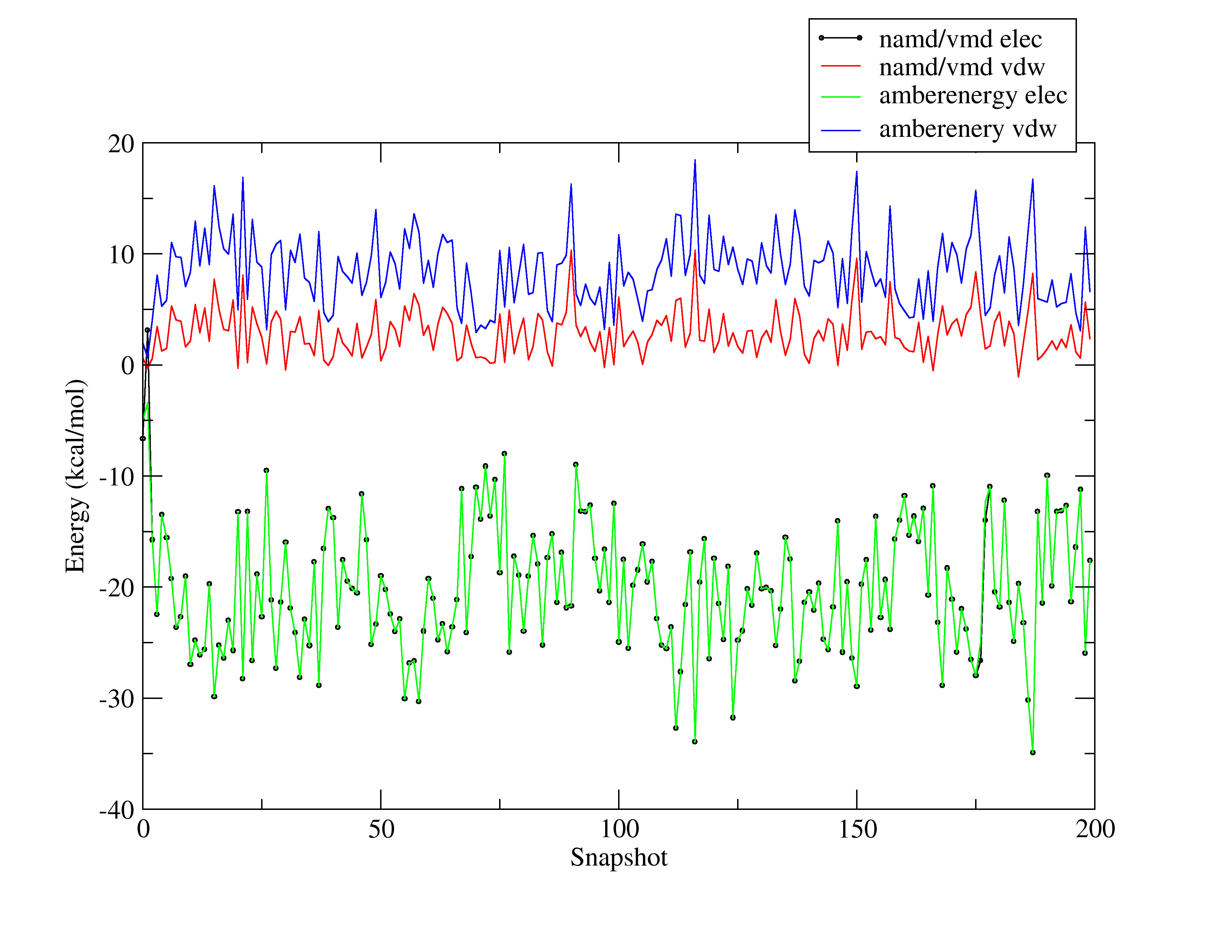Re: AMBER: interaction energies in Amber

From: Alessandro Nascimento <al.s.nascimento.gmail.com>
Date: Mon, 28 Jul 2008 18:51:01 -0600

I sent this e-mail before but didn't get it... maybe a problem with my
account...

****

Thanks, David, Carlos and Alan.

I am attaching a picture showing how different the results are.

These results were from a simulation of a waterbox (TIP3P) using
sander. I choose a waterbox to debug the contribution of the 1-4vdw
term (which is missing so far in my simple code).

The system was setup using leap as follows:

wat = createunit wat
solvatebox water TIP3PBOX 12.0

Then I minimized the system in sander, and ran a productive run
(100000 steps) also in sander, turning ntb=0.

So far, I ve being defining vdw as :

iaci = Natomtypes*(iac[i]-1);
ic = ico[(iaci+iac[j])-1]; // the `-1` corrects
for the array index which start in 0 rather than 1
if (ic > 0) {
vdw = vdw + (((LJA[ic-1])/(r2*r2*r2*r2*r2*r2))-((LJB[ic])/(r2*r2*r2))); }
else {
vdw = vdw +
(((LJA[ic-1])/(r2*r2*r2*r2*r2*r2))-((LJB[ic])/(r2*r2*r2*r2*r2))); }

Using vmd to compute the interaction energy between molecule 1 and the
other ones (using the parameters suggested in the namd manual for
amber ff simulations:

switching off
exclude 1-4scaling
1-4scaling 0.83333
cutoff 35.0

gives the results I show you in the picture

Any idea of what I am missing?

Thanks a lot,

--alessandro

-----------------------------------------------------------------------
The AMBER Mail Reflector
To post, send mail to amber.scripps.edu
To unsubscribe, send "unsubscribe amber" (in the *body* of the email)
to majordomo.scripps.edu(image/png attachment: Untitled.png)

Received on Wed Jul 30 2008 - 06:07:31 PDT
Custom Search